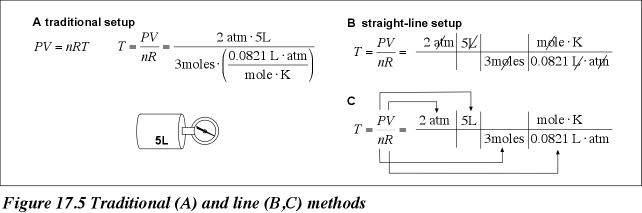# Simplifying Calculations with the Line Method

See text for other examples and solutions

Example 1: What is the approximate temperature of 3 moles of nitrogen gas contained in a 5-liter flask at 20 atmospheres (R=0.0821 L atm/mol K)?  The traditional method requires that the universal gas constant, R, be placed as a fraction in the denominator (figure 17.5A).  By contrast, the line method requires that the universal gas constant, R, be inverted and multiplied so that there are no fractions in the numerator or denominator (figure 17.5B).  It is now easier to see how units cancel (figure 17.5C).